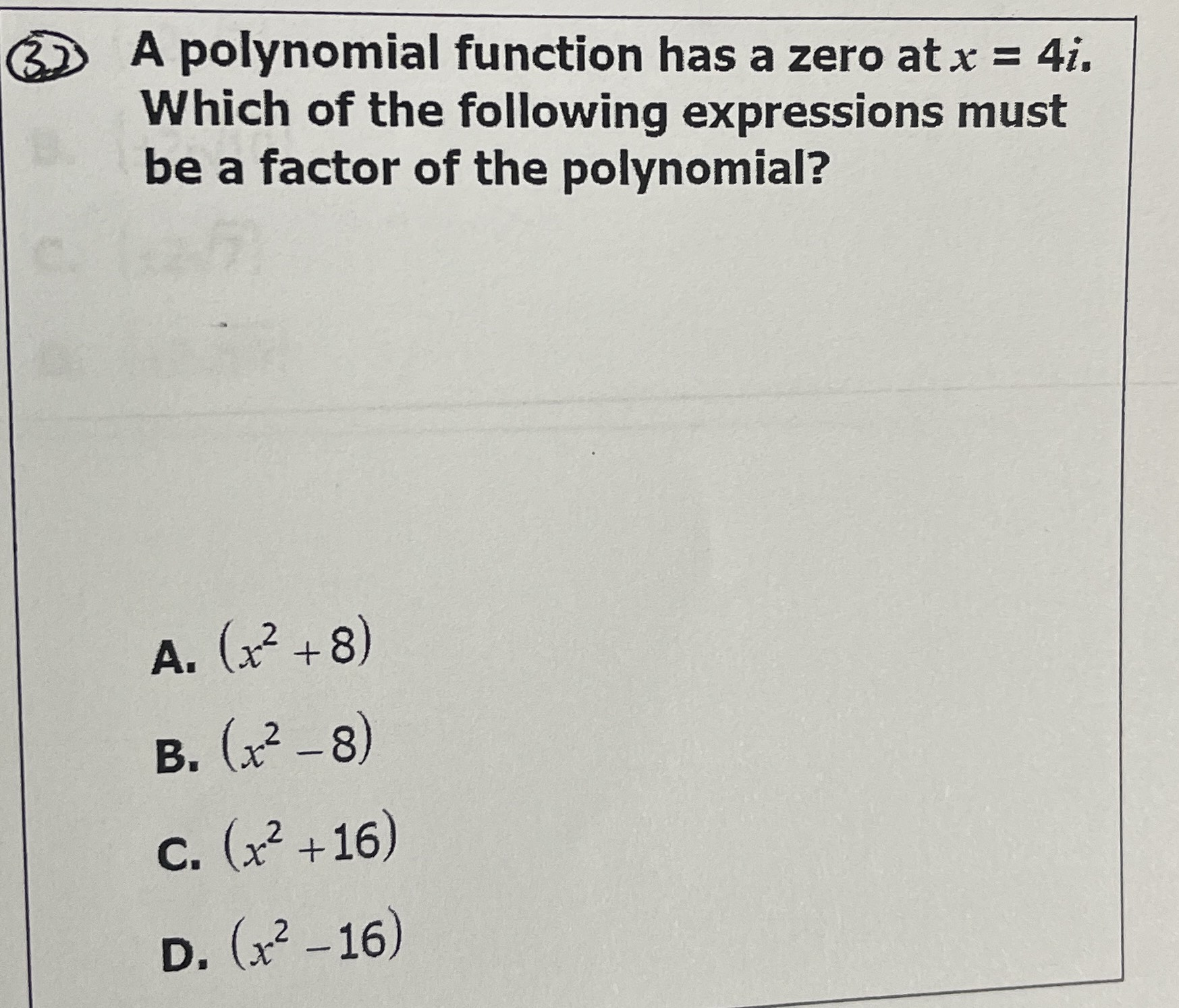### ¿Todavía tienes preguntas de matemáticas?

Pregunte a nuestros tutores expertos
Algebra
Pregunta(3) A polynomial function has a zero at $$x = 4 i$$ Which of the following expressions must be a factor of the polynomial?

A. $$( x ^ { 2 } + 8 )$$

B. $$( x ^ { 2 } - 8 )$$

C. $$( x ^ { 2 } + 16 )$$

D. $$( x ^ { 2 } - 16 )$$

$$D) x^2+ 16$$